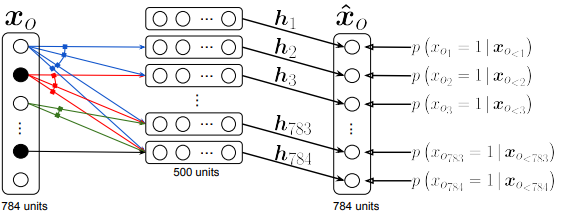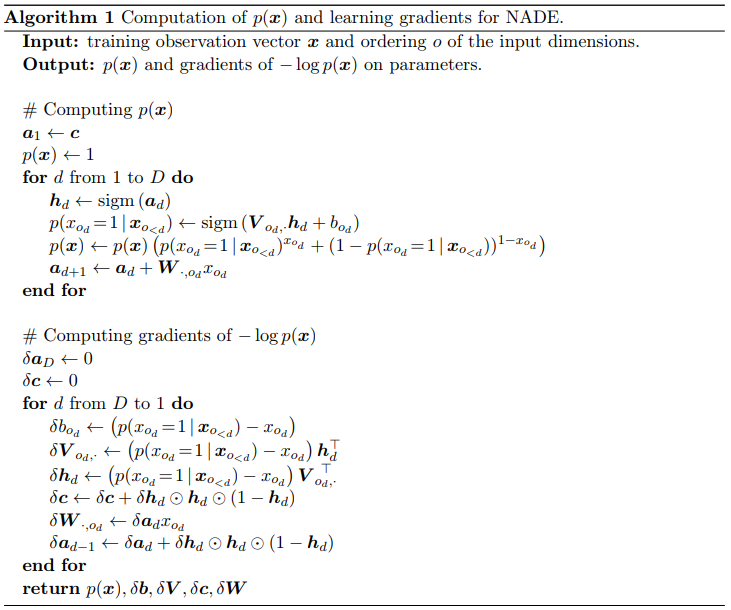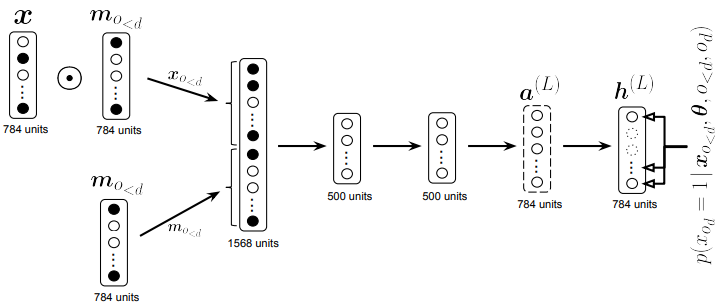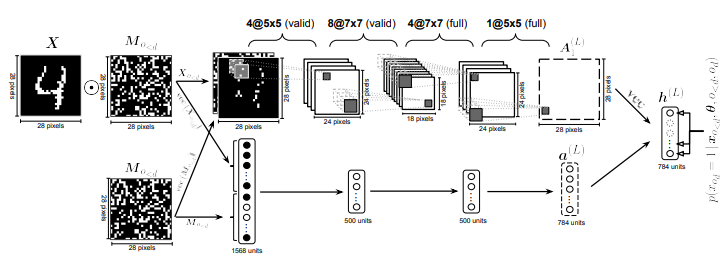## WHY?

Estimating the distribution of data can help solving various predictive taskes. Approximately three approaches are available: Directed graphical models, undirected graphical models, and density estimation using autoregressive models and feed-forward neural network (NADE).

## WHAT?

Autoregressive generative model of data consists of D conditionals. NADE use feed-forward neural network to model each conditionals. First, we assume that x is binary. W and c are shared. $% $ Computation cost is O(H) since W and c are shared. $% $ NADE can be trained by maximum likelihood. Algorithm is as follows.We can extend NADE with other options. RNADE extends binary value x to real value, estimating rhe conditionals as Gaussian mixture.Orderless deep Nade mix the order of input using binary mask and concatenate mask as input to get conditionals.ConvNADE use convolution filters as W and stacks L layers.We can combine ConvNADE with deep NADE.

## So?

This paper performed extensive experiments with NADE. NADE was able to capture fairly good distribution of MNIST.

## Critic

Autoregressive framework is nice. However, I think this can be combined with hierarchical structure of features.

Uria, Benigno, et al. “Neural autoregressive distribution estimation.” The Journal of Machine Learning Research 17.1 (2016): 7184-7220.